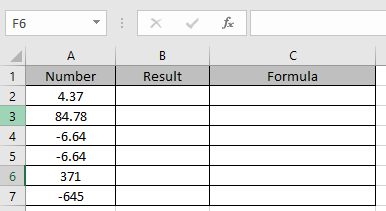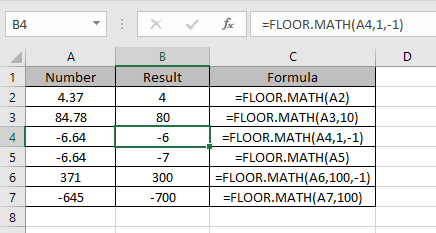# How to use the FLOOR.MATH Function in Excel

In simple words, the FLOOR.MATH function rounds down the number to the nearest specified multiple. FLOOR.MATH rounds to the nearest integer, using a 1 as significance. Positive numbers with decimal values are rounded down to the nearest integer (e.g. 6.7 is rounded down to 6) while negative numbers with decimal values are rounded away from zero (e.g. -5.8 is rounded to -6).

The FLOOR.MATH function rounds down a number to the nearest multiple.
Syntax:

=FLOOR.MATH (number, [significance], [mode])

number : number to be rounded off
significance : [optional] multiple to use when rounding. Default is 1.
mode : [optional] For rounding negative numbers toward or away from zero. Default is 0.

Let’s understand this function using it in an example.Here we will perform different operations with the numbers. So read it carefully.

Use the formula:

=FLOOR.MATH(A2)

Here significance taken as default value.
The number rounds off towards 0.

Now I have explained these numbers

=FLOOR.MATH(4.37) \\ returns 4
=FLOOR.MATH(84.78, 10) \\ returns 80
=FLOOR.MATH(-6.64, 1, -1) \\ returns -6
=FLOOR.MATH(-6.64) \\ returns -7
=FLOOR.MATH(371,100, -1) \\ returns 300
=FLOOR.MATH(-645, 100) \\ returns -700As you can see, the function rounds of the number to a specified multiple.

Hope you understood how to use FLOOR.MATH function in Excel. Explore more articles on Excel mathematical function here. Please feel free to state your query or feedback for the above article.

Related Articles:

How to use the Excel CEILING function

Rounding Numerical Calculation Results In Microsoft Excel

How to use the Excel TRUNC Function

How to Use Rounddown function in Excel

Popular Articles:

50 Excel Shortcuts to Increase Your Productivity

How to use the VLOOKUP Function in Excel

How to use the COUNTIF in Excel 2016

How to use the SUMIF Function in Excel

Terms and Conditions of use

The applications/code on this site are distributed as is and without warranties or liability. In no event shall the owner of the copyrights, or the authors of the applications/code be liable for any loss of profit, any problems or any damage resulting from the use or evaluation of the applications/code.